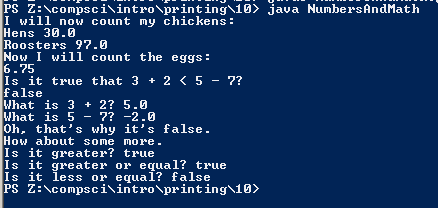# Programming Assignment #11

## Code

```  /// Name: Dylan Sleeper
/// Period: 6
/// Program Name: Numbers with math stuffs
/// File Name: NumbersAndMath.java
/// Date Finished: 9/9/2015

public class NumbersAndMath
{
public static void main( String[] args )
{

//Prints some text
System.out.println( "I will now count my chickens:" );
//Does a random math equation
System.out.println( "Hens " + ( 25.0 + 30.0 / 6.0 ) );
System.out.println( "Roosters " + ( 100.0 - 25.0 * 3.0 % 4.0 ) );

//Again just prints some text
System.out.println( "Now I will count the eggs:" );
//More math...
System.out.println( 3.0 + 2.0 + 1.0 - 5.0 + 4.0 % 2.0 - 1.0 / 4.0 + 6.0 );

//Even more text...
System.out.println( "Is it true that 3 + 2 < 5 - 7?" );
//A conditional statement that returns false
System.out.println( 3.0 + 2.0 < 5.0 - 7.0 );
//Prints some text with a math equation as well
System.out.println( "What is 3 + 2? " + ( 3.0 + 2.0 ) );
System.out.println( "What is 5 - 7? " + ( 5.0 - 7.0) );
//Sooo much printing text
System.out.println( "Oh, that's why it's false." );

System.out.println( "How about some more." );
//3 conditionals: The first and second are true while the third is false.
System.out.println( "Is it greater? " + ( 5.0 > -2.0 ) );
System.out.println( "Is it greater or equal? " + ( 5.0 >= -2.0 ) );
System.out.println( "Is it less or equal? " + ( 5.0 <= -2.0 ) );
}
}

```

## Outputs